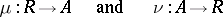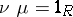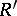# Retract

(diff) ← Older revision | Latest revision (diff) | Newer revision → (diff)

of an object of a category

A concept generalizing the corresponding concepts in algebra and topology. An objectof a categoryis called a retract of an objectif there exist morphismssuch that. The morphismin this case is a monomorphism and, moreover, the equalizer of the pair of morphisms. Dually, the morphismis an epimorphism and also the co-equalizer of the pair of morphisms.is sometimes known as a section andas a retraction.

Ifis a retract of an objectand an objectis isomorphic to, thenis a retract of. Therefore an isomorphism class of retracts forms a single subobject of. Each retract of, defined by morphismsand, corresponds to an idempotent morphism. Two retractsandof an objectbelong to the same subobject if and only if they correspond to the same idempotent. The retracts of any object of an arbitrary category form a set.